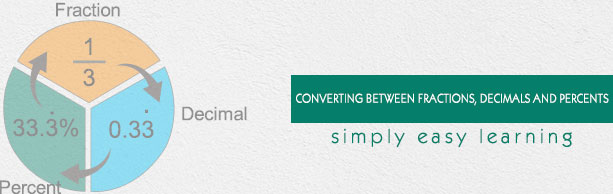Converting Between Fractions, Decimals, & Percents

This tutorial provides comprehensive coverage of Converting between fractions, decimals and percents based on Common Core (CCSS) and State Standards and its prerequisites. Students can navigate learning paths based on their level of readiness. Institutional users may customize the scope and sequence to meet curricular needs. This simple tutorial uses appropriate examples to help you understand Converting between fractions, decimals and percents in a general and quick way.

Audience

This tutorial has been prepared for beginners to help them understand the basics of Converting between fractions, decimals and percents. After completing this tutorial, you will find yourself at a moderate level of expertise in Converting between fractions, decimals and percents, from where you can advance further.

Prerequisites

Before proceeding with this tutorial, you need a basic knowledge of elementary math concepts such as number sense, addition, subtraction, multiplication, division, whole numbers, fractions, decimals, comparing and ordering whole numbers, ratios, rates and percents and so on.